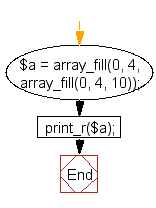﻿ PHP Array Exercise: Create a two-dimensional array - w3resource# PHP Array Exercises : Create a two-dimensional array

## PHP Array: Exercise-56 with Solution

Write a PHP script to create a two-dimensional array (4x4), initialized to 10.

Note: Use array_fill() function.

Sample Solution:

PHP Code:

``````<?php
\$a = array_fill(0, 4, array_fill(0, 4, 10));
print_r(\$a);
?>
```
```

Sample Output:

```Array
(
 => Array
(
 => 10
 => 10
 => 10
 => 10
)

 => Array
(
 => 10
 => 10
 => 10
 => 10
)

 => Array
(
 => 10
 => 10
 => 10
 => 10
)

 => Array
(
 => 10
 => 10
 => 10
 => 10
)

)
```

Flowchart:PHP Code Editor:

What is the difficulty level of this exercise?

﻿

## PHP: Tips of the Day

PHP: Extract numbers from a string

```\$str = 'In My Cart : 11 12 items';
preg_match_all('!\d+!', \$str, \$matches);
print_r(\$matches);
```